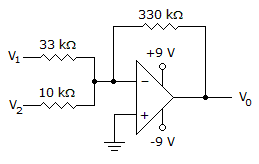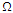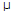# Electronic Devices - Op-Amp Applications

Exercise : Op-Amp Applications - General Questions
26.
Calculate the output voltage if V1 = –3.3 V and V2 = 0.8 V.0 V
6.6 V
–4 V
2 V
Explanation:

Vo = -Rf(V1/R1 + V2/R2)

27.
When a number of stages are connected in parallel, the overall gain is the product of the individual stage gains.
True
False
Explanation:
No answer description is available. Let's discuss.

28.
Calculate the cutoff frequency of a first-order low-pass filter for R1 = 2.5 kand C1 = 0.05F.
1.273 kHz
12.73 kHz
127.3 kHz
127.30 Hz
Explanation:
No answer description is available. Let's discuss.

29.
A filter that provides a constant output from dc up to a cutoff frequency and passes no signal above that frequency is called a ________ filter.
low-pass
high-pass
bandpass
Explanation:
No answer description is available. Let's discuss.

30.
Calculate the output voltage if V1 = 0 V and V2 = 0.2 V.0 V
–6.6 V
–4 V
2 V